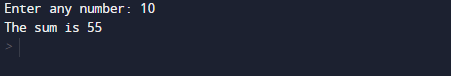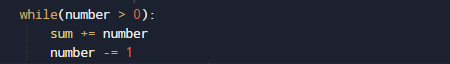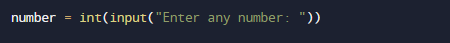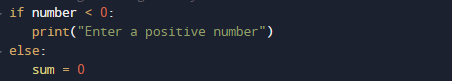# Python Program to Find the Sum of Natural Numbers

In this tutorial you will learn about the Python Program to Find the Sum of Natural Numbers and its application with practical example.

In this tutorial, we will learn to create a Python program to Find the Sum of Natural Numbers    using Python programming.

## Prerequisites

Before starting with this tutorial we assume that you are best aware of the following Pyhton programming topics:

• Basic Input and Output
• Basic Python  programming.
• Python if-else statements.
• Python Loop.

## What Is Natural  Number?

Simply all the counting numbers start from {1, 2,3.4…} are commonly called Natural numbers.Natural numbers  include all the positive integers from 1 to infinity (∞).

## Python Program to Find the Sum of Natural Numbers

In this program we will Find the Sum of Natural Numbers.Firstly we declare required variable and also initiates required values in variable. Next we take value from user at run time and then after we will find the sum of all natural number to given number.

## OutputIn our program while will run until  all numbers adds all numbers to the variable Sum (sum+=number).and after calculating value we will print sum or all natural number.In the above program, we have first declared and initialized a set variables required in the program.

• number=to take value from user.
• sum = for counting sum of numbers.

In the above program, we have first declared and initialized a set variables required in the program. In our  program first we will take a value from user.And after that we will check the given number is valid or notand after that we will calculate sum of all digit between given number.Within the loop, we add all values in variable Sum(sum+=number) .After calculating values  of all natural number between the given numbers natural number.At last we will Print sum of the all Natural number given by user.

In this tutorial we have learn about the Python Program to Find the Sum of Natural Numbers and its application with practical example. I hope you will like this tutorial.